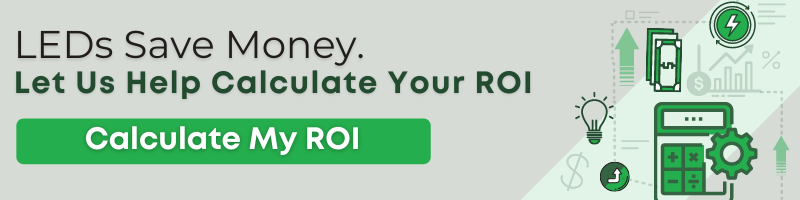Are you interested in an energy efficient lighting solution for your organization, business, or facility?

Picking the most efficient lighting starts with a thorough understanding of energy terminology as it relates to electricity. The four big measures you need to be aware of are Joules, Watts, Watt-Hours, and Lumens/Watt. We'll break them down one at a time below.

## Joules (J):

Joules are the SI (International System of Units) unit of energy. They are defined as 1 Joule = 1 kg·m2/s2. If you’re concerned with energy efficiency in lighting, what you’re really trying to do is to minimize the total number of Joules you consume while maximizing the effective light output.

## Watts (W) and Kilowatts (kW):

Watts are the SI unit of power. Kilowatts are equivalent to 1,000 Watts and are the most frequently used unit of electrical power. Power in general is defined as energy over time. Watts are defined as 1 Watt = 1 Joule per second (1W = 1 J/s) which means that 1 kW = 1000 J/s. A Watt is the amount of energy (in Joules) that an electrical device (such as a light) is burning per second that it’s running. So a 60W bulb is burning 60 Joules of energy every second you have it turned on. In return, you get a particular amount of light, also known as luminance. For more on luminance and an even more important measure (foot-candles), read here.

Example: If a room has one hundred 40W light bulbs running at once, you are using 4000 Watts (4000 Joules/second). Imagine getting the same (or better) lighting quality by using one hundred 5W lights instead. Now you’re only burning 500 watts. That’s only 12.5% as much energy as you were using before; a savings of 87.5%!

## Watt-Hours (Wh) and Kilowatt-Hours (kWh):

Imagine you have your 60 Watt bulb on for an entire hour. Enter a new, and sometimes confusing term, “Watt-hours.” Since you ran a 60 Watt bulb for 1 hour, you have used 60 Watt-hours of energy (not power). If the owner of a large building (such as a factory or real estate development) is trying to minimize their energy footprint, they want to minimize the number of Watt-hours they are burning throughout a given 24 hour period. This is the same thing as saying they want to minimize the number of Joules they are consuming; it is simply a different, more common unit of measurement. 1 Watt-hour is equal to 3600 Joules (3.6kJ). One Kilowatt-Hour is the same as 1,000 Watt-hours and is the most common unit used in electrical energy consumption.

## Lumens/Watt:

This is a unit of measurement for a term called luminous efficacy (you can read about it in detail here). Luminous efficacy is a quantity that describes the amount of illumination (lumens) that you get for a given amount of power (watts). All else being equal, you want to maximize lumens while minimizing the power required to produce them.

Different devices require different amounts of power to make them run. In addition, different devices emit a different quantity and quality of light for a given power setting. Other major considerations include off-the-shelf price, lifespan, and maintenance/replacement costs. For a more in-depth discussion on the most energy efficient lighting solutions, visit us at Stouch Lighting or try our ROI calculator. We’re here to help!